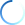SAVE
\$19.99
1st Edition

# Introductory Mathematical Analysis for Quantitative Finance

By

,

## Giulia Spaletta

• Available for pre-order. Item will ship after April 16, 2020
ISBN 9780815372547
April 16, 2020 Forthcoming by Chapman and Hall/CRC
310 Pages - 22 B/W Illustrations

SAVE ~ \$19.99
was \$99.95
USD \$79.96

Prices & shipping based on shipping country

## Book Description

Introductory Mathematical Analysis for Quantitative Finance is a textbook designed to enable students with little knowledge of mathematical analysis to fully engage with modern quantitative finance. A basic understanding of dimensional Calculus and Linear Algebra is assumed.

The exposition of the topics is as concise as possible, since the chapters are intended to represent a preliminary contact with the mathematical concepts used in Quantitative Finance. The aim is that this book can be used as a basis for an intensive one-semester course.

Features:

• Written with applications in mind, and maintaining mathematical rigor.
• Suitable for undergraduate or master's level students with an Economics or Management background.
• Complemented with various solved examples and exercises, to support the understanding of the subject.

Euclidean space.  Sequences and series of functions.  Multidimensional differential calculus.  Ordinary differential equations of first order: methods for explicit solutions. Linear differential equations of second order.  Prologue to Measure theory.  Lebesgue integral.  Radon-Nikodym theorem.  Multiple integrals. Gamma and Beta functions. Fourier ransform on the real line. Parabolic equations.

## Author(s)

### Biography

Daniele Ritelli is associate professor of mathematical analysis at the Department of Statistical Sciences of the University of Bologna, in Italy. He teaches ordinary and partial differential equations and special functions in various three-year and magister degree courses in statistics and finance. His research interests concern special functions, ordinary differential equations and pure and applied mathematics.

Giulia Spaletta is associate professor of numerical analysis at the Department of Statistical Sciences of the University of Bologna, in Italy. She lectures in various three-year and master degree courses in computer science, statistics and economics. Her research interests include numerical error analysis in linear algebra and differential equations and numerical modeling in biomedical engineering.

## Reviews

Introductory Mathematical Analysis for Quantitative Finance is one of those rare textbooks designed to introduce upper undergraduates or beginning graduate students in Economics and Management to the indispensable analytic tools needed for approaching the technicalities of Mathematical Finance. The text assumes a minimum level of knowledge in Advanced Calculus and Linear Algebra and leads the reader to the first notions of Measure Theory and Fourier transform to arrive at the solution of the Black Scholes equation. The book is very well written and is perfect for students studying Economics and Management.

—Vincenzo Vespri, Università degli Studi di Firenze